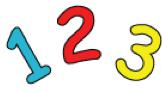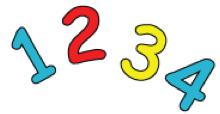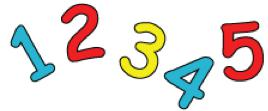#### You may also like### Pebbles

Place four pebbles on the sand in the form of a square. Keep adding as few pebbles as necessary to double the area. How many extra pebbles are added each time?### Bracelets

Investigate the different shaped bracelets you could make from 18 different spherical beads. How do they compare if you use 24 beads?### Sweets in a Box

How many different shaped boxes can you design for 36 sweets in one layer? Can you arrange the sweets so that no sweets of the same colour are next to each other in any direction?

# Curious Number

##### Age 7 to 11Challenge LevelAre you curious about numbers? Can you use your mathematical skills to find some solutions to the problems below?

Can you order the digits 1, 2 and 3 to make a number which is divisible by 3?
And when the final digit is removed again it becomes a two-digit number divisible by 2,
then finally a one-digit number divisible by 1?

Can you order the digits 1, 2, 3 and 4 to make a number which is divisible by 4?
And when the final digit is removed it becomes a three-digit number which is divisible by 3.
And when the final digit is removed again it becomes a two-digit number divisible by 2,
then finally a one-digit number divisible by 1?

Can you order the digits 1, 2, 3, 4 and 5 to make a number which is divisible by 5?
And when the final digit is removed it becomes a four-digit number which is divisible by 4.
And when the final digit is removed it becomes a three-digit number which is divisible by 3.
And when the final digit is removed again it becomes a two-digit number divisible by 2,
then finally a one-digit number divisible by 1?

What systems are you using?
What do you know about numbers which can be divided by 3, 4, 5?
Now what about taking this further for digits 1, 2, 3, 4, 5, and 6?
What do you know about numbers which can be divided by 6, 7, 8 and 9?

You might now like to have a go at the problem Dozens.